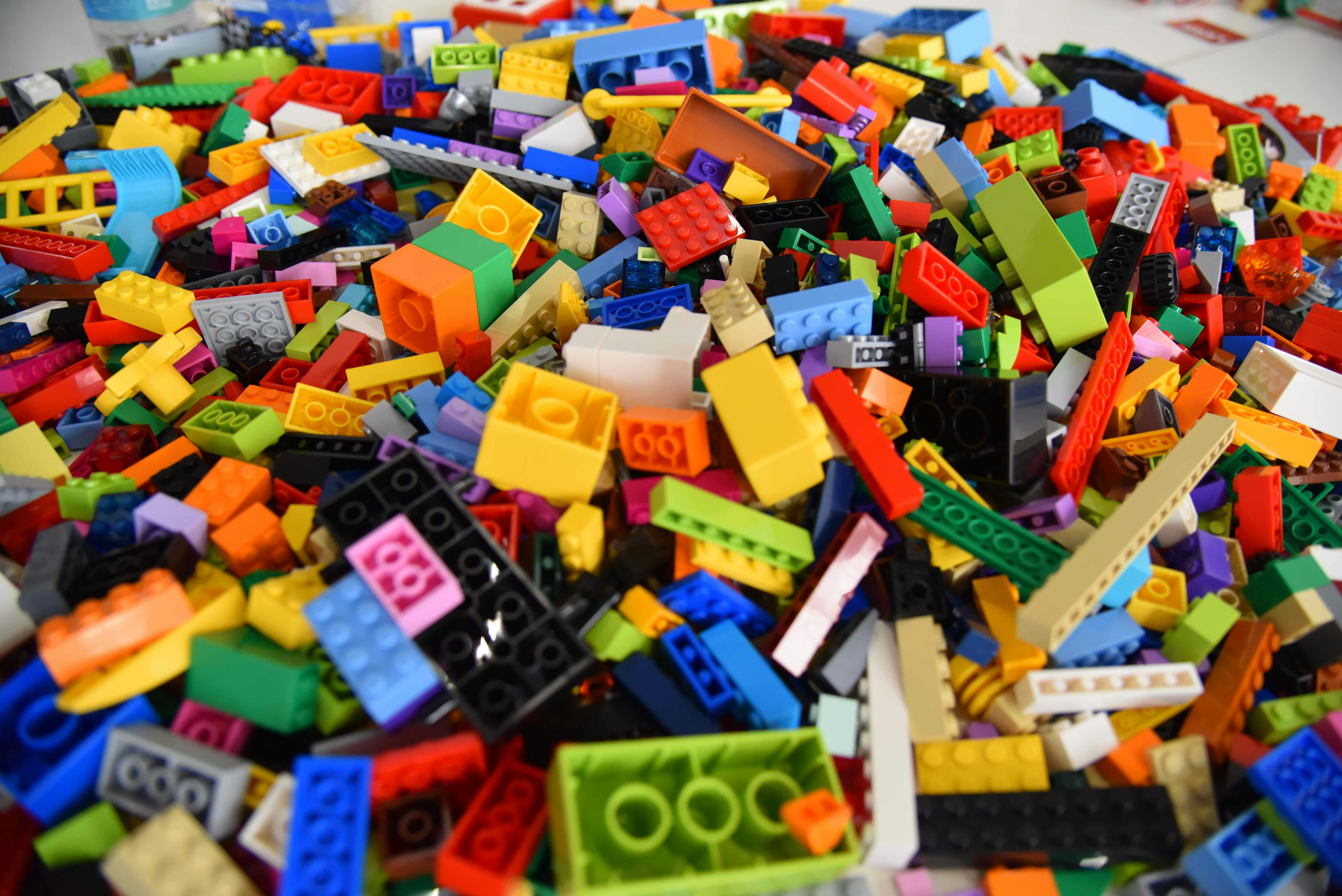MACS VR Concepts
Share
Explore#Math Blocks

Math blocks are 3 dimensional representations of mathematical components. This includes concepts like integers, variables, operators, and entire functions and equations. These math blocks can we pieced together and rearranged like Legos to allow for a simplified and intuitive way to interact with math.
Goal:
Allow students to focus on learning high level math concepts without worrying/being distracted by all the math principals that came before.
Lean on the familiarity of legos and puzzles to explain how certain math concepts fit together to produce larger functions.
Allow students to visualize and interact with math concepts in 3 dimensions.
Simplify the math learning process without making it as easy as a multiple choice question.
Create more immersive, high quality visual aids for mathematical concepts.
Visuals
Interactive Experience Flow
3D representation

Features:
Math Blocks
Math blocks are 3 dimension representations of mathematical components, this includes integers, variables, operators, and entire functions and equations. These math blocks can we pieced together and rearranged to like Legos to allow for a simplified and intuitive way to interact with math.
Simplify wand
Sometimes the common step of simplifying an equation can remove the student from the mathematical concept they were just learning, as they now have to think back to old math concepts and rules to simplify the equation before returning to where they were in that lesson and trying to remember the new concept they were trying to learn. In order to ease this friction during the learning of a new concept we can give the students a Simplify wand that does some of the simplification of larger problems without the user losing sight of the new concept they were trying to learn.
As the user advances the wand will become unavailable
The student still needs to understand when a problem requires simplification and the desired section that need to be simplified in order to get the correct outcome. So while we are reducing the friction of simplifying while learning new math concepts, we are still reinforcing the order of operations a student must know to complete these types of problems.
This idea of providing a tool to ease the friction of certain mathematical processes while learning a new concept can be extended to a myriad of other mathematical processes. The Simplify wand just seemed like a good place to start.
Step by Step Guided Instruction
Larger mathematical concepts and problems will be broken down into a step by step process where we validate the users understanding at each step before they can progress. This will allow the student to get feedback at every step of the process and for us to provide a deeper explanation on sections where the student needs it. This process allows us to move at the pace of the student rather than requiring them to keep up with a video.
Animated and interactive visual aids
Instead of simple line drawings or still images, our math problems would be represented my three dimensional visual aids that will animate and build upon itself as the user goes through the step by step instructions. These aids will also be interactive so students can manipulate the visual to see how changes in the represented problem may occur. See the mock up below for an example of interactivity.
Click on sections of the problem to see how the visual aid changes as you progress through the problem.
Teaching Scenarios:
Give the users a bunch of blocks and tell them to build an equation that can provide the desired outcome with a specific input.
Give students a completed equation made of blocks with one piece missing and tell them to fill it in.
Step by step guided instruction through a series of equations in block form.
Inspiration / Reference Material
Function Builder is a web based game that has a similar form of interaction to what we want with the Math blocks.